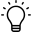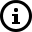# Product Cost and Profit Reports

Report Toaster has introduced Product Cost into our dataset, which allows us to calculate gross profit.

Shopify allows product cost, or cost of goods sold (COGS), to be tracked at the product SKU or variant level.When cost is used in combination with the sales price for a product, the gross profit can be calculated. A few terms to be familiar with in order to understand profit calculations:

Net Quantity: The quantity of units sold less items returned for a particular product.For example, if a customer bought 3 of Item A and then returned 1, then the net quantity (calculated as 3 - 1) is 2.

Cost: The total cost of the units sold for a specific product.For example, if the product cost of Item A is \$5 and the net quantity is 2, then the cost (calculated as 5 * 2) is \$10.

Net Sales: The amount of sales minus refunds and discounts.For example, if the price of Item A is \$11 and the net quantity sold is 2, then the net sales (calculated as 11 * 2) is \$22.

Gross Profit: The amount of profit generated from the sale of a specific product. The profit is calculated as net sales less cost.For example, if the net sales of Item A is \$22 and the cost is \$10, then the gross profit (calculated as 22 - 10) is \$12.

Gross Profit Margin: The profit margin on the sale of a specific product. The margin is calculated as ([net sales - cost] / net sales) * 100.For example, if your net sales are \$22 and your cost is \$10, then the gross margin (calculated as ([22 - 10] / 22) * 100) is 55%.

Unfortunately, Shopify reports do not track product cost at a specific point in time. Instead, if the product cost is updated for a specific SKU, all Shopify reports will calculate profit based on the current cost. Shopify recommends using a reporting app from the Shopify app store to report profits at a specific point in time, and Report Toaster is one of those apps!
All of our Profit reports calculate using the cost at the time of an order, making them accurate at each point in time.

See below for a list of profit reports available.

• Profit by Collection
• Profit by Product
• Profit by Product Variant
• Profit by Product Vendor
• Profit by Sales Channel
• Cost of Goods Sold

###Suggestion Box

Didn't find what you were looking for in our knowledge base? Click here to suggest an article, and we'll try to put something together for you.